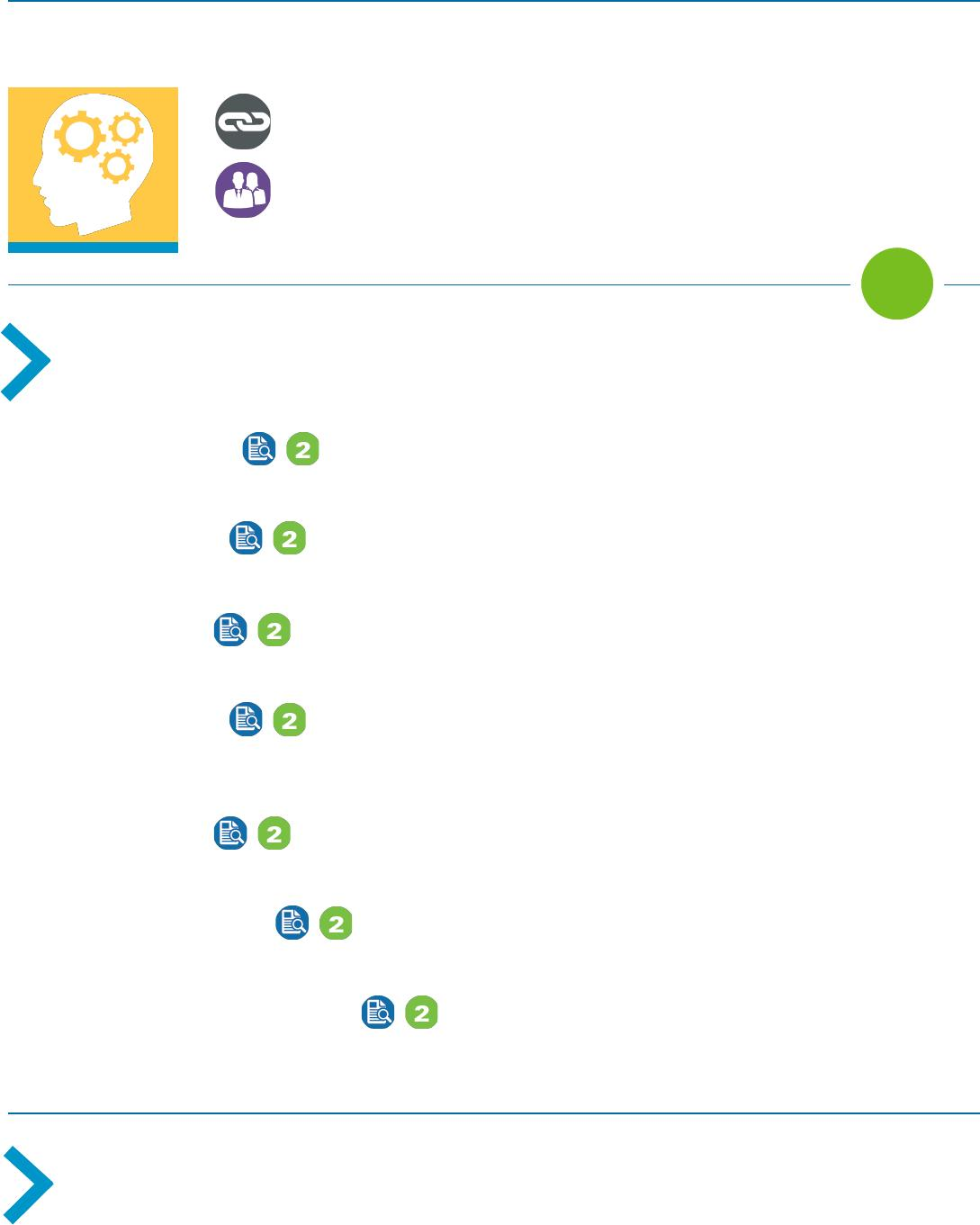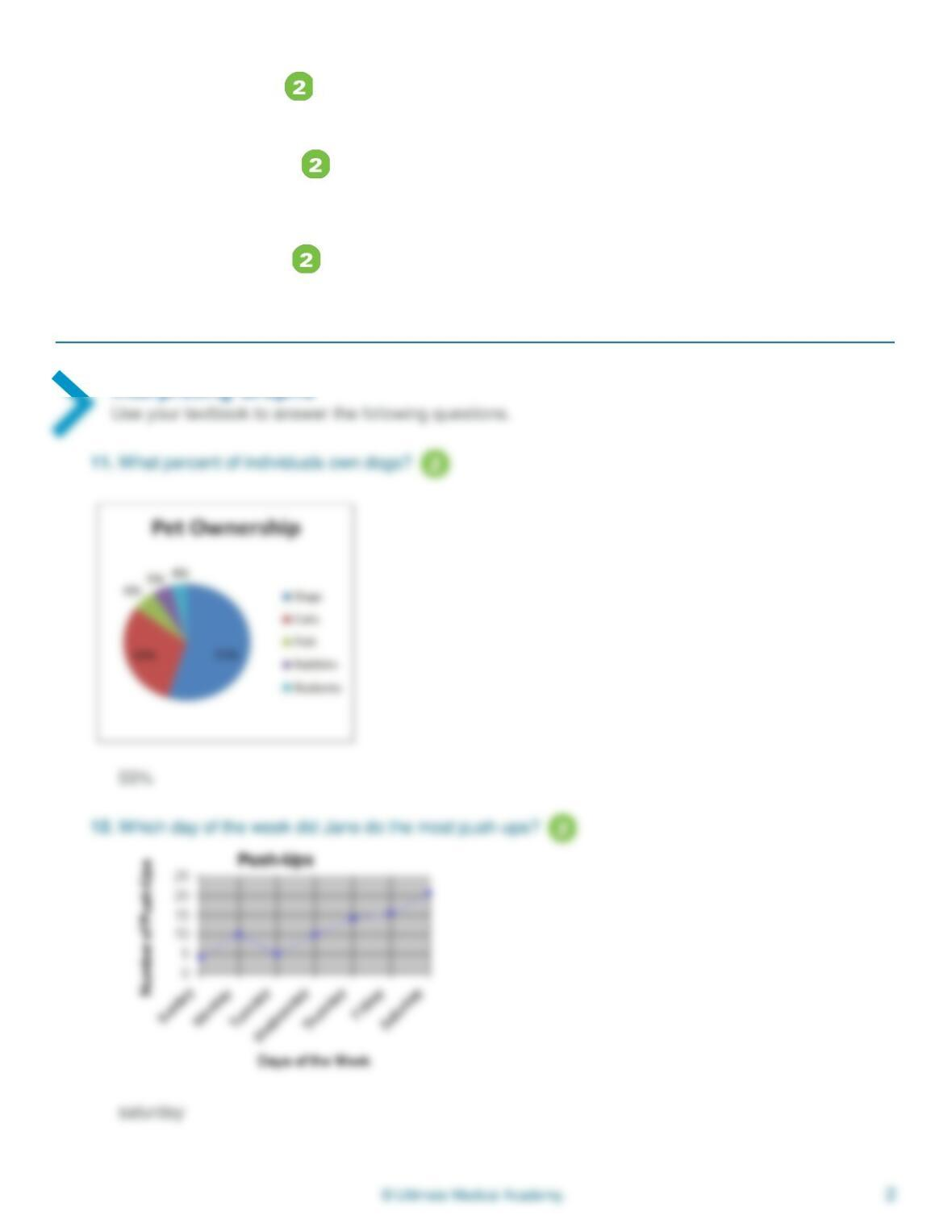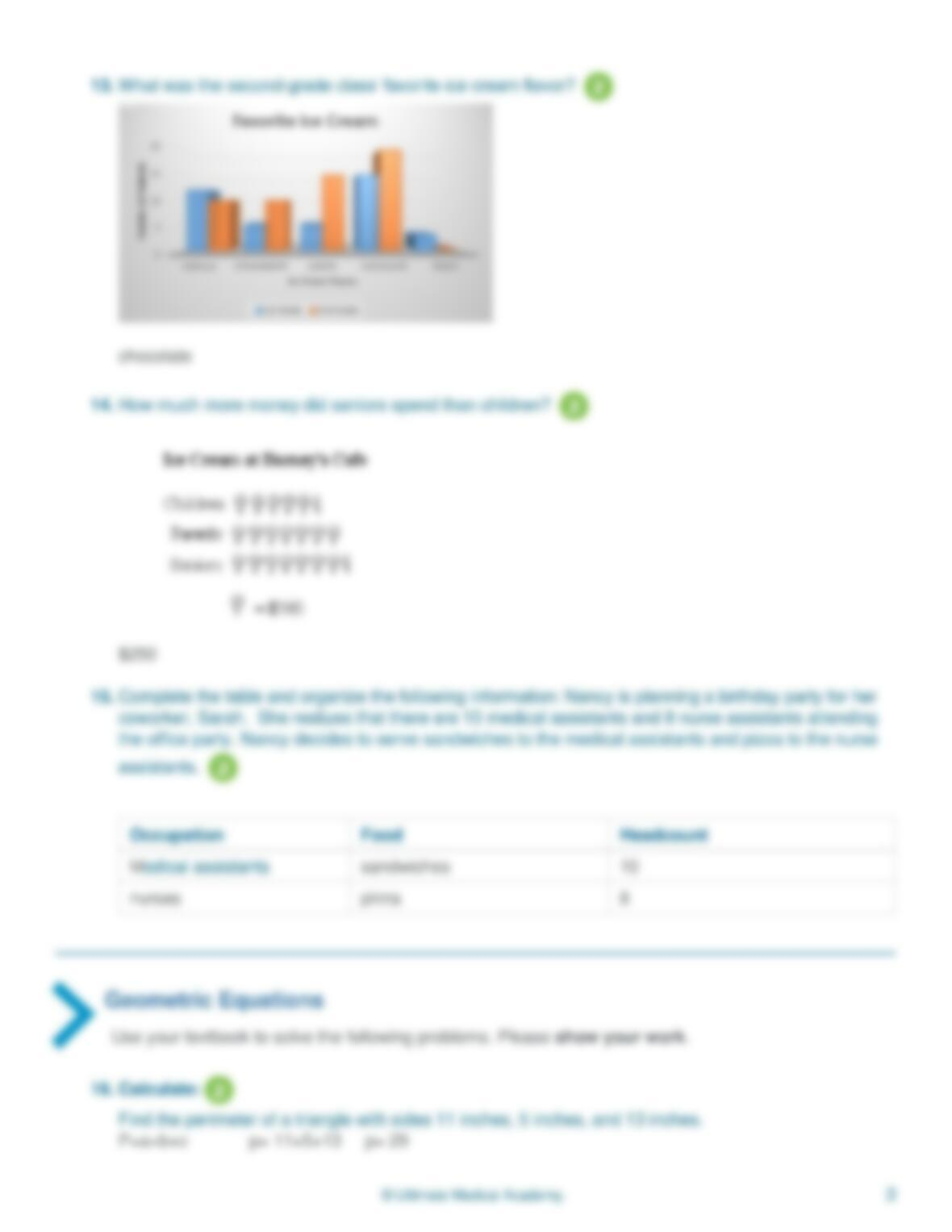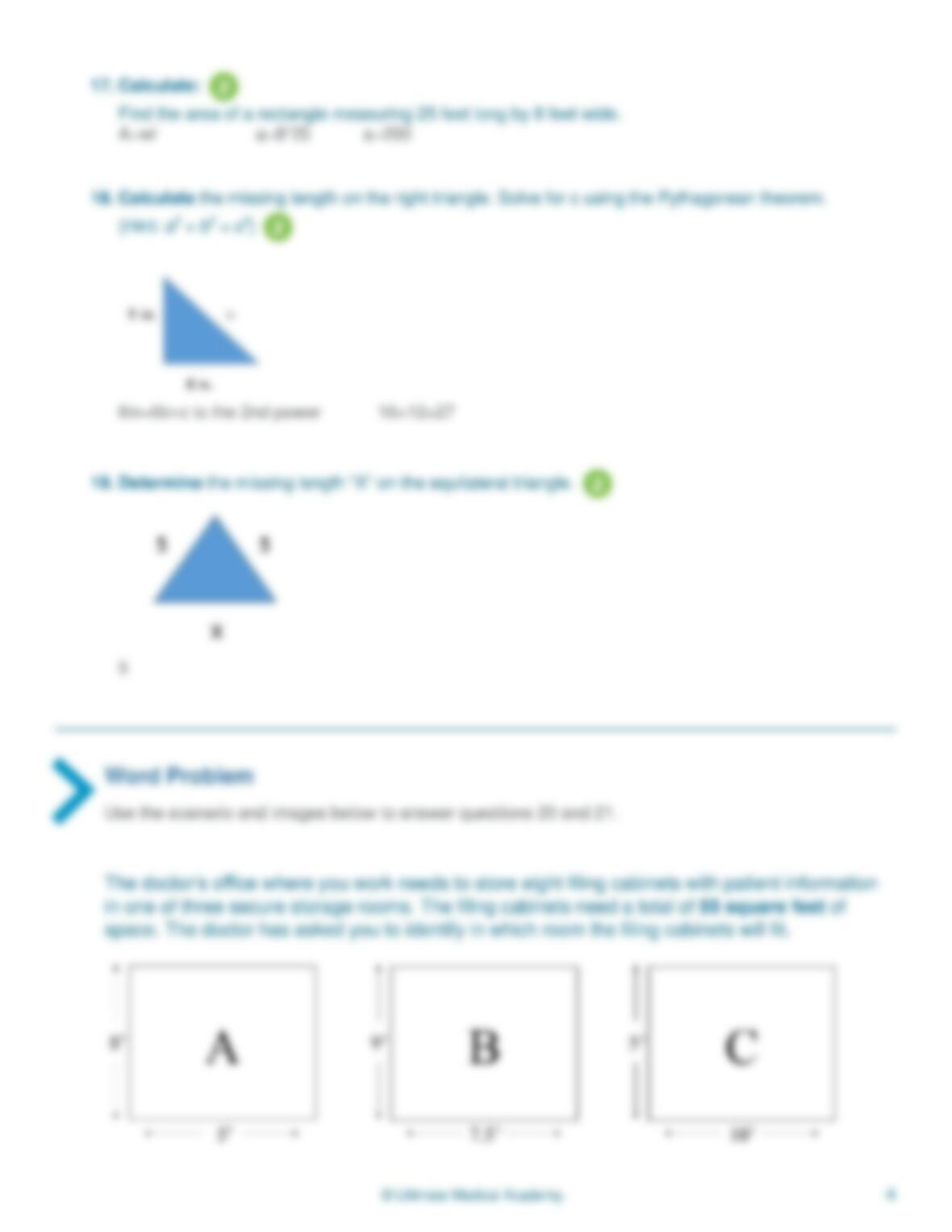Type
Essay
Pages
5 pages
Word Count
621 words
School
Course Code
math

# Math

November 21, 2020MA1015: Week 5 Assignment
1
What are statistics and geometry
and how will I use these concepts in my healthcare career?
This assignment helps you apply your knowledge from this week’s modules
Employers in the healthcare industry expect employees to have
mathematical knowledge and skills to perform tasks such as properly
managing data, records, inventory, and prescriptions.
Mathematical Vocabulary
Use chapters 7 and 12 of your textbook to define the following vocabulary.
1. Define: Statistics
The branch of mathematics concerned with data,or numerical information.
2. Define: Graphs
A display that provides a pictorial representation of data
3. Define: Mean
The sum of all values divided by the number of those values also known as the average value
4. Define: Median
The value that separates a list of values in such a way that there is the same number of values below
the median as above
5. Define: Mode
In a set of numbers the value that occurs most frequently
6. Define: Square Root
A square root of a number is one or two identical factors of that number
7. Define: Pythagorean Theorem
The square of the hypotenuse of a right triangle is equal to the sum of the squares of the two legs
Statistical Calculations
use this dataset for the next three questions: 5, 10, 12, 4, 6, 11, 13, 5
508. Calculate the mean.
4+5+5+6+10+11+11+12+13= 66 66/8=8.25
9. Calculate the median.
6+10= 16 16/2= 8
10. Determine the mode.
The mode is 5
Interpreting Graphs